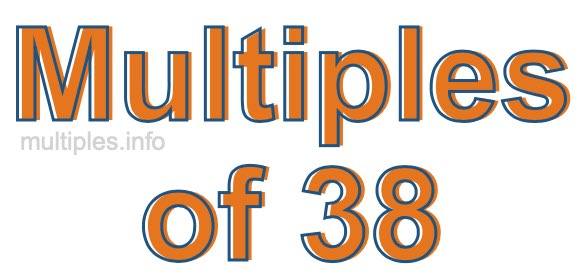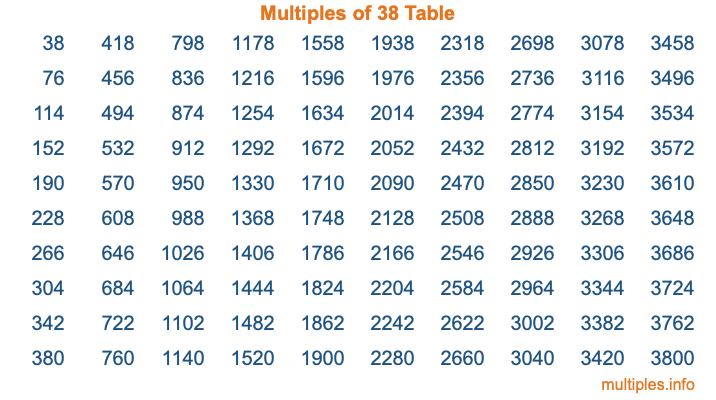Multiples of 38Welcome to the Multiples of 38 page. Here we will first teach you everything you will ever need to know about the multiples of 38, and then give you a study guide summary of everything we taught you to make sure you remember it all. Use this page to look up facts and learn information about the multiples of 38. This page will make you a multiples of thirty-eight expert!

Definition of Multiples of 38
Multiples of 38 are all the numbers that when divided by 38 equal an integer. Each of the multiples of 38 are called a multiple. A multiple of 38 is created by multiplying 38 by an integer.

Therefore, to create a list of multiples of 38, you start with 1 multiplied by 38, then 2 multiplied by 38, then 3 multiplied by 38, and so on for as long as you want. Thus, the list of the first five multiples of 38 is 38, 76, 114, 152, and 190. To see a larger list of multiples of 38, see the printable image of Multiples of 38 further down on this page. We also have a category where you can choose any nth multiple of 38.

Multiples of 38 Checker
The Multiples of 38 Checker below checks to see if any number of your choice is a multiple of 38. In other words, it checks to see if there is any number (integer) that when multiplied by 38 will equal your number. To do that, we divide your number by 38. If the the quotient is an integer, then your number is a multiple of 38.

Is  a multiple of 38?

Least Common Multiple of 38 and ...
A Least Common Multiple (LCM) is the lowest multiple that two or more numbers have in common. This is also called the smallest common multiple or lowest common multiple and is useful to know when you are adding our subtracting fractions. Enter one or more numbers below (38 is already entered) to find the LCM.

Check out our LCM Calculator if you need more details about the Least Common Multiple or if you need the LCM for different numbers for adding and subtraction fractions.

nth Multiple of 38
As we stated above, 38 is the first multiple of 38, 76 is the second multiple of 38, 114 is the third multiple of 38, and so on. Enter a number below to find the nth multiple of 38.

th multiple of 38

Multiples of 38 vs Factors of 38
38 is a multiple of 38 and a factor of 38, but that is where the similarities end. All postive multiples of 38 are 38 or greater than 38. All positive factors of 38 are 38 or less than 38.

Below is the beginning list of multiples of 38 and the factors of 38 so you can compare:

Multiples of 38: 38, 76, 114, 152, 190, etc.

Factors of 38: 1, 2, 19, 38

As you can see, the multiples of 38 are all the numbers that you can divide by 38 to get a whole number. The factors of 38, on the other hand, are all the whole numbers that you can multiply by another whole number to get 38.

It's also interesting to note that if a number (x) is a factor of 38, then 38 will also be a multiple of that number (x).

Multiples of 38 vs Divisors of 38
The divisors of 38 are all the integers that 38 can be divided by evenly. Below is a list of the divisors of 38.

Divisors of 38: 1, 2, 19, 38

The interesting thing to note here is that if you take any multiple of 38 and divide it by a divisor of 38, you will see that the quotient is an integer.

Multiples of 38 Table
Below is an image of the first 100 multiples of 38 in a table. The table is in chronological order, column by column. The first column has the first ten multiples of 38, the second column has the next ten multiples of 38, and so on.The Multiples of 38 Table is also referred to as the 38 Times Table or Times Table of 38. You are welcome to print out our table for your studies.

Negative Multiples of 38
Although not often discussed or needed in math, it is worth mentioning that you can make a list of negative multiples of 38 by multiplying 38 by -1, then by -2, then by -3, and so on, to get the following list of negative multiples of 38:

-38, -76, -114, -152, -190, etc.

Multiples of 38 Summary
Below is a summary of important Multiples of 38 facts that we have discussed on this page. To retain the knowledge on this page, we recommend that you read through the summary and explain to yourself or a study partner why they hold true.

There are an infinite number of multiples of 38.

A multiple of 38 divided by 38 will equal a whole number.

38 divided by a factor of 38 equals a divisor of 38.

The nth multiple of 38 is n times 38.

The largest factor of 38 is equal to the first positive multiple of 38.

38 is a multiple of every factor of 38.

38 is a multiple of 38.

A multiple of 38 divided by a divisor of 38 equals an integer.

38 divided by a divisor of 38 equals a factor of 38.

Any integer times 38 will equal a multiple of 38.

Multiples of a Number
Here you can get the multiples of another number, all with the same attention to detail as we did for multiples of 38 on this page.

Multiples of
Multiples of 39
Did you find our page about multiples of thirty-eight educational? Do you want more knowledge? Check out the multiples of the next number on our list!